# Instrumentation Diagrams Multiple Choice Questions

Instrumentation Diagrams Multiple Choice Questions

Question 1

Identify what type of diagram this is: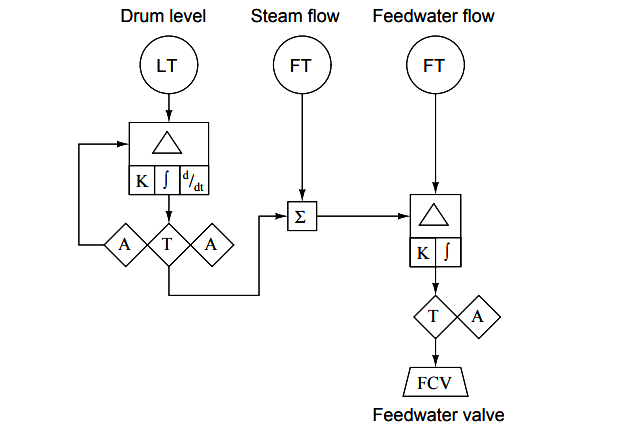(A) Wiring diagram
(B) Isometric drawing
(C) SAMA diagram
(D) P&ID
(E) Loop diagram

Question 2

Identify what type of diagram this is: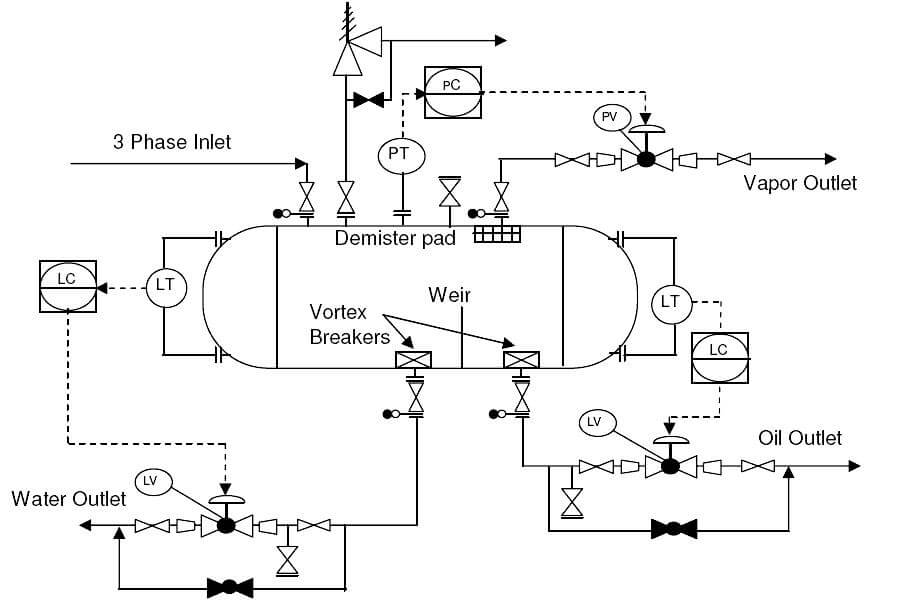(A) Wiring diagram
(B) Isometric drawing
(C) Loop diagram
(D) P&ID
(E) SAMA diagram

Question 3

According to this diagram, the controller is , and it is located :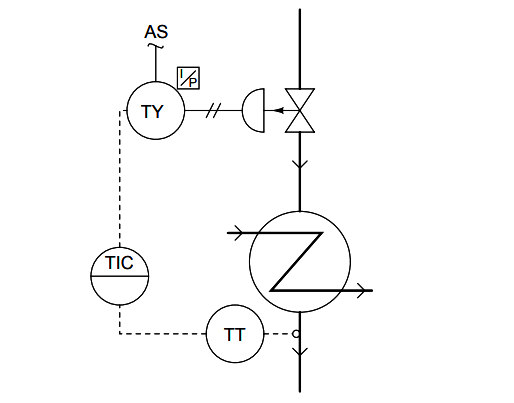(A) Pneumatic, in the field (process) area
(B) Pneumatic, on the front of a secondary control panel
(C) Pneumatic, on the front of the main control panel
(D) Electronic, behind the main control panel
(E) Electronic, on the front of the main control panel

Question 4

Identify the following instrumentation (P&ID and loop diagram) line types, from left to right: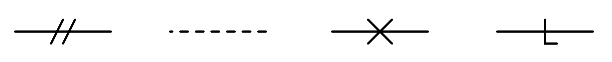(A) pneumatic, electric, capillary, hydraulic
(B) electric, pneumatic, digital network, filled system
(C) pneumatic, electric, hydraulic, mechanical link
(D) electric (on/off), electric (analog), pneumatic, digital network
(E) pneumatic, mechanical link, hydraulic, capillary

Question 5

P&ID stands for ?

(A) Process & Information diagram
(B) Process & Instrumentation diagram
(C) Piping & Information drawings
(D) Piping & Instrumentation diagram

Question 6

If the gas flow through FT-42 is 1200 SCFM, how much electric current is going through cable 23 and through cable 27 ?(A) ICBL23 = 14.2 mA , ICBL27 = 14.2 mA
(B) ICBL23 = 16.8 mA , ICBL27 = 16.8 mA
(C) ICBL23 = 16.8 mA , ICBL27 = 18.3 mA
(D) ICBL23 = 16.8 mA , ICBL27 = 14.2 mA
(E) ICBL23 = 14.2 mA , ICBL27 = 16.8 mA

Question 7

Calculate the voltage between terminals 15 and 16 of TB27 when the measured flow rate is 106 GPM.
Assume a loop power supply voltage of exactly 24.00 volts, and negligible wire resistance:(A) 26.70 volts
(B) 22.28 volts
(C) 21.30 volts
(D) 24.00 volts
(E) 2.696 volts

Question 8

If the thermocouple fails open, what will happen?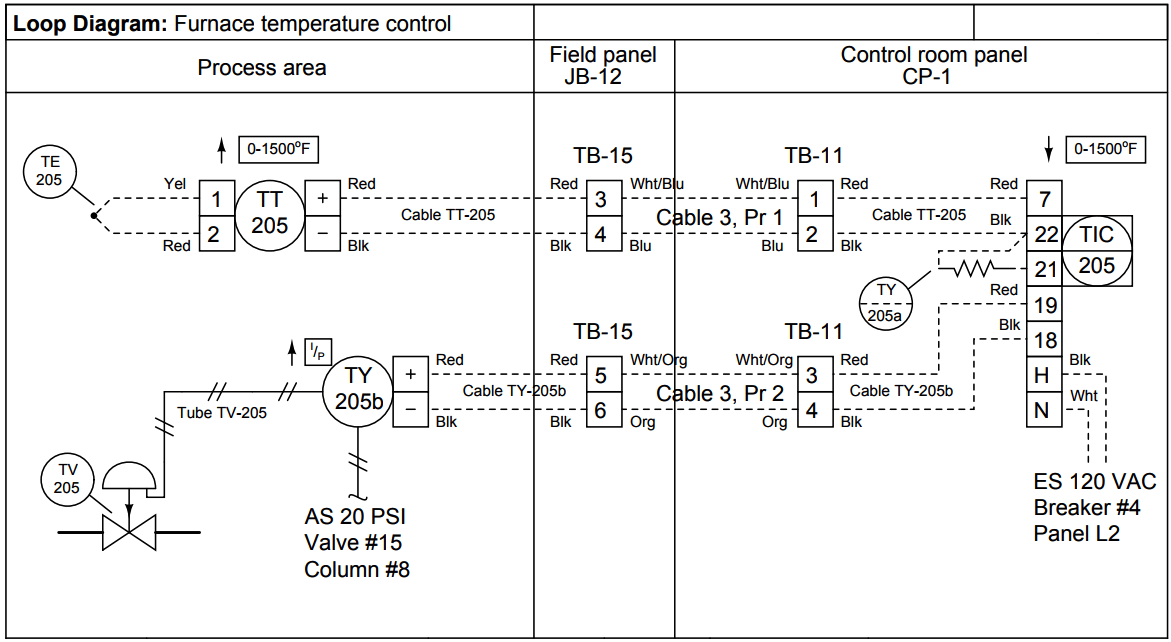(A) Valve TV-205 will hold position, stabilizing the process
(B) Valve TV-205 will close shut, making the process cool down
(C) Valve TV-205 will close shut, making the process warm up
(D) Valve TV-205 will open wide, making the process cool down
(E) Valve TV-205 will open wide, making the process warm up

Question 9

What kind of device provides the setpoint for the analytical indicating controller in this diagram?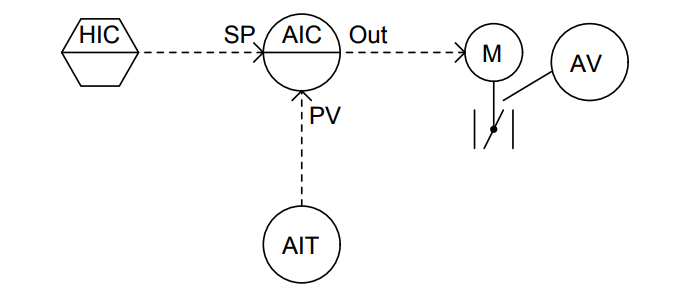(A) A Workstation
(B) A mathematical relay
(C) Another panel-mounted controller
(D) A programmable logic controller (PLC)
(E) A pneumatic hand station

Question 10

What sort of alarm function is provided by the controller in this diagram?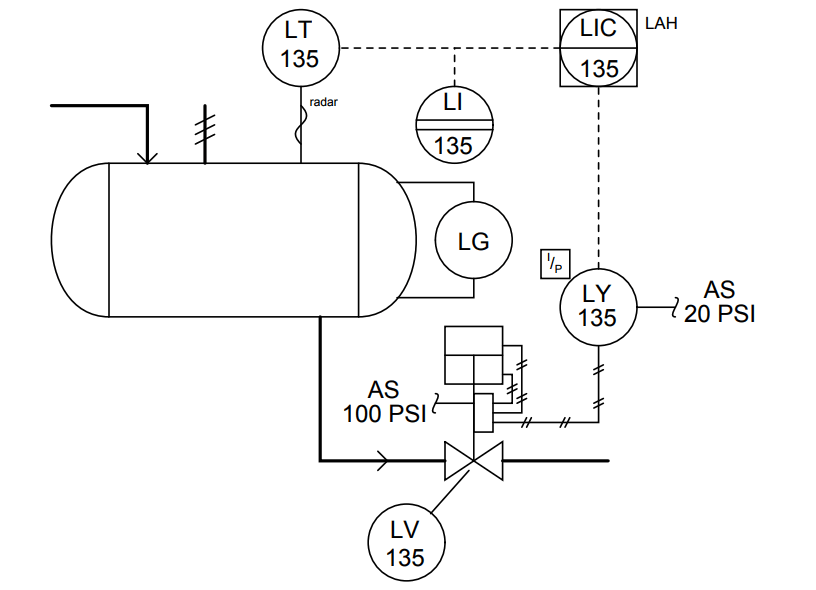(A) Low-flow alarm
(B) High-temperature alarm
(C) Low-level alarm
(D) High-flow alarm
(E) High-level alarm

Credits : by Tony R. Kuphaldt

Be the first to get exclusive content straight to your email.
We promise not to spam you. You can unsubscribe at any time.

### 4 thoughts on “Instrumentation Diagrams Multiple Choice Questions”

1.Question 6
You should have someone check the answer given.

Question 7
You are missing at least one piece of information to be able to arrive at an answer.

2.I agree with john question 6 answer is not correct , information is also not complete for question 6 & 7

3.Question 6:
I23 = 80%.16+4 = 16.8mA
I27 = Sqrt(80%).16+ 4 = 18.3 mA

So C is the correct answer not E ? Am I right ?

4.